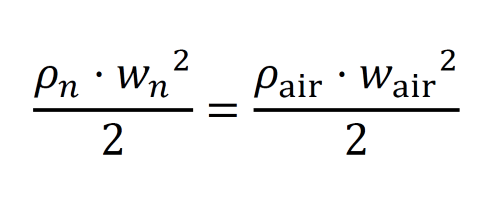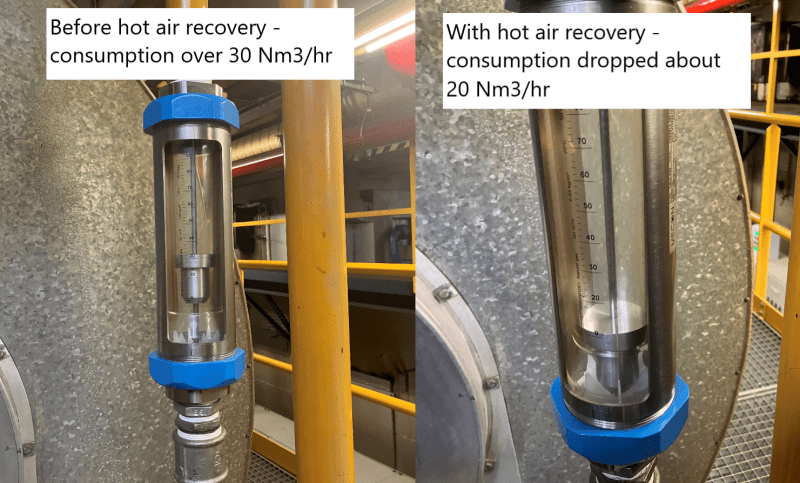×
INTELLIGENT WORK FORUMS
FOR ENGINEERING PROFESSIONALS

Are you an
Engineering professional?
Join Eng-Tips Forums!
• Talk With Other Members
• Be Notified Of Responses
• Keyword Search
Favorite Forums
• Automated Signatures
• Best Of All, It's Free!

*Eng-Tips's functionality depends on members receiving e-mail. By joining you are opting in to receive e-mail.

#### Posting Guidelines

Promoting, selling, recruiting, coursework and thesis posting is forbidden.

# Mass flow of air from volumetric flow

## Mass flow of air from volumetric flow

(OP)
Hi everyone,

I tried looking for something similar, but I have not found it.

I have measured the airflow (air speed) in the round ducting using a pitot tube and I need to find out the mass flow of it. At some point I must be making a mistake because when I calculate it from normal cubic meters per hour (Nm3/hr) I got a different value than when I calculate it from the actual flow (before converting to normal cubic meters).

Normal cubic meters are when gas is at:
tn= 0 deg Celsius (=273.15K)
pn = 1013,25 mbar.
densn 1.293 kg/m3 - density of air at normal conditions

d = 250mm - diameter of duct
A = 0.049m2(cross sectional area of the duct)
tair = 336°C (= 609K) - temperature of air in the duct
dair = 0.580 kg/m3 - density of air in the duct

pd = 0,825 mbar (= 82,5 Pa) - measured average dynamic pressure
w = 16,87 m/s - average air speed calculated from dynamic pressure ad velocity

Actual volumetric flow is:
f = w* A = 16,87 m/s * 0,049 m3= 0,828 m3/hr = 2981 m3/hr

To calculate the flow at normal conditions i first calculate the airspeed at these conditions. Assuming that in the duct the static pressure is equal to normal pressure
wn = w* sqrt (dair/dn) = 11,296 m/s

Flow at normal condition is
fn = wn* A = 0,55 Nm3/s = 1996 Nm3/hr

All of that seems easy but there must be an arror as when I calculate the mass from actual and normal flow I have different results

from actual flow
m = f * dair = 2981 * 0,580 = 1728 kg/h

from normalized flow
m = fn * dn = 1157 kg/h

The mass flow in kilograms should always be the same.

In the atatchment there is an excel file with the same calculations. I don't know where the dfference comes from. I calculated the compressibility factor (Z) for both states (actual and normal) but it is very close to 1, so that's not it. Can anybody direct me to the solution.

Regards,
Marcin

### RE: Mass flow of air from volumetric flow

Why sqrt(dair/dn)?

Shouldn't this just be the ratio without the square root?

Also the European comma instead of decimal point confuses me and you seem to use both, so density is 1.293, but velocity is 11,296??, not 11.296.

Also I highly doubt the 336C air is at exactly 101365 Pa. Even a small difference will make a big impact.

Then from your calc I get 1726 kg/hr.

But your original calc is wrong. fn * Dn should be 2580 kg/hr. I think you multiplied the normal air flow by the density at 300C....

Remember - More details = better answers
Also: If you get a response it's polite to respond to it.

### RE: Mass flow of air from volumetric flow

(OP)
Thank you for the reply. Sorry about the coma/dot errors. I pasted it from excel and then edited it manually to make it easier for non-Europeans.

You are of course right abot the incorrectly calculated density. I attach the corrected excel file. This comes from the Bernulli's equation with the assumption that head is 0 (horizontal duct) and the static pressure is equal to normal pressure.

ps+pd+ρ⋅g⋅h=constWhen I calculate it from the gas law, then it becomes simple. p*V / T = const

Vn = Vair * (Pair/Pn) * (Tn/Tair) * (Zn/Zair)

for simplicity Z1 = Z2 = 1

With that the flow is 1337 Nm3/hr which gives mass flow of 1728 kg/h

The question is, why Bernullis equation gives incorrect answer, but it is Probably because the change of static pressure is not considered. So in order to apply the Bernullis principle I should first always measure the actual static pressure in the duct. Right?

In that case the welocity of air recalculated to normal conditions would be:Any thoughts on that? I would really appreciate some feedback from a person who does shuch measurements /calculations for living.

### RE: Mass flow of air from volumetric flow

Hi,
Review the basic equations:
P*V=Z*R*T real Gas law > Qv1/Qv2= Z1*T1*P2/(Z2*T2*P1); Qv =volumetric flowrate; T, P absolute values.
1, conditions T1, P1; 2, condition (T2, P2)
Mass conservation > Qv1/Qv2= ρ2/ρ1
> As the size of the pipe is the same V1/V2=ρ2/ρ1; V = velocity
No Square root
you may want to review how the velocity is calculated using a pitot tube:

https://www.brighthubengineering.com/hydraulics-ci...
Note: Bernoulli
v1= 11.245 m/s; v2=25.11 m/s
q1=0.552 m3/s; q2=1.2326m3/s
m1=m2 =0.7109 kg/s
ρ1=1.2879 kg/m3;ρ2=0.5768 kg/m3
Z1=Z2 # 1; T1= 273.15 K, P1 = P2 = 1013 mbar, T2=t2+273.15;
EOS:PR
note: You may have some moisture in air.

Good luck
Pierre

### RE: Mass flow of air from volumetric flow

(OP)
Thank you Pierre. I think that now I have the right way of calculatng the flow in normal cubic meters and in kilograms per hour. I will have to follow the standard of measuring with the pitot tube, when possible (usuallly there is only 1 opening for the measuring tube)

There is another issue though, maybe connected to the moisture in air. Different duct, different condition. There is a new installation of hot air recovery from one of the oven to the another. Using hot air instead of fresh air allowes to save on gas consumption. These are lacquering ovens (they extract the solvent from the lacquer and then cure it)

d= 250mm -> A = 0,096m2
trec = 291°C - Temperature of exhaust gas in the duct
tamb - 31 °C - fresh air intake

pd - 0,2116 mbar - Average dynamic pressure (several sets of measurements)
w - 8,22 m/s - average air velocity in the duct

F = 0,791 m/s = 2848 m3/h = 1783 kg/h - flow of pre-heated air in the duct
Fn = 1379 Nm3/h - flow at normal conditions

cp = 1026 J / kg * K - specyfic heat of air

Using the pre-heated air at 291°C instead of fresh air at 31 °C in theory is equivalent of energy saving of 476 MJ/h or 132 kW.

cgas = 35,17 MJ/m3 - calorific value of natural gas declared by supplier

In theory this should save usin 13,522 m3/ h of natural gas, but in fact this is around 20 ( I can see it directly on the rotameter for natural gus before the burner). The difference was instatanous and remained. Also the indication on the rotaeters was stable before and after the hot air recovery on. The humidty of the air was around 80 % at that day (temp inside 30 °C, outside 15 °C ). How can I add this humidity to the heat capacity of air?So the results are way better than in the calculation, but for the future I need to know how to calculate it properly.

BTW It is not my project, I joined when it was already starting up.

Regards,
Marcin

### RE: Mass flow of air from volumetric flow

Hi,
Basically, you have to perform mass and Heat balances to calculate the improvement using hot air.
Note: I'm not sure that your Rota meters are the best technology for measuring your consumption of NG. You should perform your balances over a period of time to reduce the discrepancies, let say a day, a week, a month, the best way being the comparison of the monthly bill before and after the change.
Good luck
Pierre

### RE: Mass flow of air from volumetric flow

(OP)
Thank you for the support. I will try to calculate it properly. On Thursday I measurre the airflows again. Then I see if the results are consistent with the precious ones

#### Red Flag This Post

Please let us know here why this post is inappropriate. Reasons such as off-topic, duplicates, flames, illegal, vulgar, or students posting their homework.

#### Red Flag Submitted

Thank you for helping keep Eng-Tips Forums free from inappropriate posts.
The Eng-Tips staff will check this out and take appropriate action.

#### Resources

Low-Volume Rapid Injection Molding With 3D Printed Molds
Learn methods and guidelines for using stereolithography (SLA) 3D printed molds in the injection molding process to lower costs and lead time. Discover how this hybrid manufacturing process enables on-demand mold fabrication to quickly produce small batches of thermoplastic parts. Download Now
Examine how the principles of DfAM upend many of the long-standing rules around manufacturability - allowing engineers and designers to place a partâ€™s function at the center of their design considerations. Download Now
Industry Perspective: Education and Metal 3D Printing
Metal 3D printing has rapidly emerged as a key technology in modern design and manufacturing, so itâ€™s critical educational institutions include it in their curricula to avoid leaving students at a disadvantage as they enter the workforce. Download Now

Close Box

# Join Eng-Tips® Today!

Join your peers on the Internet's largest technical engineering professional community.
It's easy to join and it's free.

Here's Why Members Love Eng-Tips Forums:

•Talk To Other Members
• Notification Of Responses To Questions
• Favorite Forums One Click Access
• Keyword Search Of All Posts, And More...

Register now while it's still free!